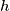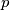IMO Shortlist 1960 problem 7

Kvaliteta:
Avg: 0,0
Težina:
Avg: 0,0
An isosceles trapezoid with bases$a$ and$c$ and altitude$h$ is given.

a) On the axis of symmetry of this trapezoid, find all points$P$ such that both legs of the trapezoid subtend right angles at$P$;

b) Calculate the distance of$p$ from either base;

c) Determine under what conditions such points$P$ actually exist. Discuss various cases that might arise.
Izvor: Međunarodna matematička olimpijada, shortlist 1960